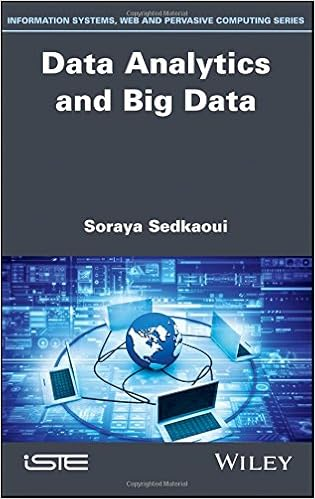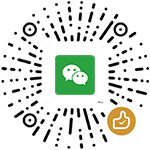# Data Analytics and Big DataData Analytics and Big Data
by Soraya Sedkaoui
pages 页数： 220 pages
Publisher Finelybook 出版社： Wiley-ISTE; 1 edition (July 24,2018)
Language 语言： English
ISBN-10 书号： 1786303264
ISBN-13 书号： 9781786303264
Part 1. Towards an Understanding of Big Data:
Chapter 1. From Data to Big Data:
You Must Walk Before You Can Run
1.1. Introduction.
1.2. No analytics without data
1.2.2. Raw data
1.2.4. Images,audios and videos.
1.2.5. The Internet of Things.
1.3. From bytes to yottabytes: the data revolution
1.4. Big data: definition
1.5. The 3Vs model.
1.6. Why now and what does it bring?.
1.7. Conclusions.
Chapter 2. Big Data: A Revolution that 2.1. Introduction.
2.2. Beyond the 3Vs.
2.3. From understanding data to knowledge.
2.4. Improving decision-making.
2.5. Things to take into account 2.5.1. Data complexity
2.5.2. Data quality: look out! Not all data are the right data 2.5.3. What else?. Data security.
2.6.1. Opportunities.
2.6.2. Challenges
2.7. Conclusions.
Part 2. Big Data Analytics: A Compilation of Advanced Analytics Techniques that Covers a Wide Range of Data
Chapter 3. Building an Understanding of Big Data Analytics
3.1. Introduction.
3.2. Before breaking down the process.
What is data analytics?
3.3. Before and after big data analytics.
What is the difference?
3.6. New statistical and computational paradigm within the big data context
3.7. Conclusions.
Chapter 4. Why Data Analytics and When Can We Use It?.
4.1. Introduction.
4.2. Understanding the changes in context.
4.3. When real time makes the difference.
4.4. What should data analytics address?.
4.5. Analytics culture within companies
4.6. Big data analytics application: examples.
4.7. Conclusions
Chapter 5. Data Analytics Process:
There's Great Work Behind the Scenes
5.1. Introduction.
5.2. More data,more questions for better answers.
5.2.1. We can never say it enough: "there is no good wind for those who don't know where they are going".
5.2.2. Understanding the basics: identify what we already know and what we have yet to find out.
5.2.3. Defining the tasks to be accomplished.
5.2.5. Understanding data analytics is good but knowing how to use it is better!(What skills do you need?)
5.2.6. What does the data project cost and how will 5.2.7. What will it mean to you once you find out?
5.3. Next steps: do you have an idea about a "secret sauce"?
5.3.1. First phase: find the data(data collection)
5.3.2. Second phase: construct the data(data preparation).
5.3.3. Third phase: go to exploration and modeling 5.3.4. Fourth phase: evaluate and interpret the results (evaluation and interpretation).
5.3.5. Fifth phase: transform data into actionable knowledge (deploy the model).
5.4. Disciplines that support the big data analytics process.
5.4.1. Statistics
5.4.2. Machine learning
5.4.3. Data mining.
5.4.4. Text mining.
5.4.5. Database management systems
5.4.6. Data streams management systems
5.5. Wait,it's not so simple: what to avoid when building a model?
5.5.1. Minimize the model error
5.5.2. Maximize the likelihood of the model 5.5.3. What about surveys?
5.6. Conclusions.
Part 3. Data Analytics and Machine Learning:
the Relevance of Algorithms
Chapter 6. Machine Learning:
a Method of Data Analysis that Automates
Analytical Model Building
6.1. Introduction
6.2. From simple descriptive analysis to predictive and
prescriptive analyses: what are the different steps?
6.3. Artificial intelligence: algorithms and techniques
6.4. ML: what is it?
6.5. Why is it important?
6.6. How does ML work?.
6.6.1. Definition of the business need(problem statement)
and its formalization.
6.6.2. Collection and preparation of the useful data that will be
used to meet this need.
6.6.3. Test the performance of the obtained model.
6.6.4. Optimization and production start
6.7. Data scientist: the new alchemist
6.8. Conclusion
Chapter 7. Supervised versus Unsupervised Algorithms:
a Guided Tour
7.1. Introduction.
7.2. Supervised and unsupervised learning.
7.2.1. Supervised learning: predict,predict and predict!
7.2.2. Unsupervised learning: go to profiles search!.
7.3. Regression versus classification
7.3.1. Regression
7.3.2. Classification
7.4. Clustering gathers data.
7.4.1. What good could it serve?.
7.4.2. Principle of clustering algorithms.
7.4.3. Partitioning your data by using the K-means algorithm
7.5. Conclusion
Chapter 8. Applications and Examples
8.1. Introduction.
8.2. Which algorithm to use?.
8.2.1. Supervised or unsupervised algorithm:
in which case do we use each one
8.2.2. What about other ML algorithms?
8.3. The duo big data/ML: examples ofuse
8.3.1. Netflix: show me what you are looking at and I' ll
personalize what you like.
8.3.2. Amazon: when AI comes into your everyday life.
8.3.3. And more: proof that data are a source of creativity

## 下载地址：

Data Analytics and Big Data 9781786303264.epub

## 下载地址：

Data Analytics and Big Data 9781786303264.pdf

### 评论 抢沙发

#### 支付宝扫一扫打赏#### 微信扫一扫打赏